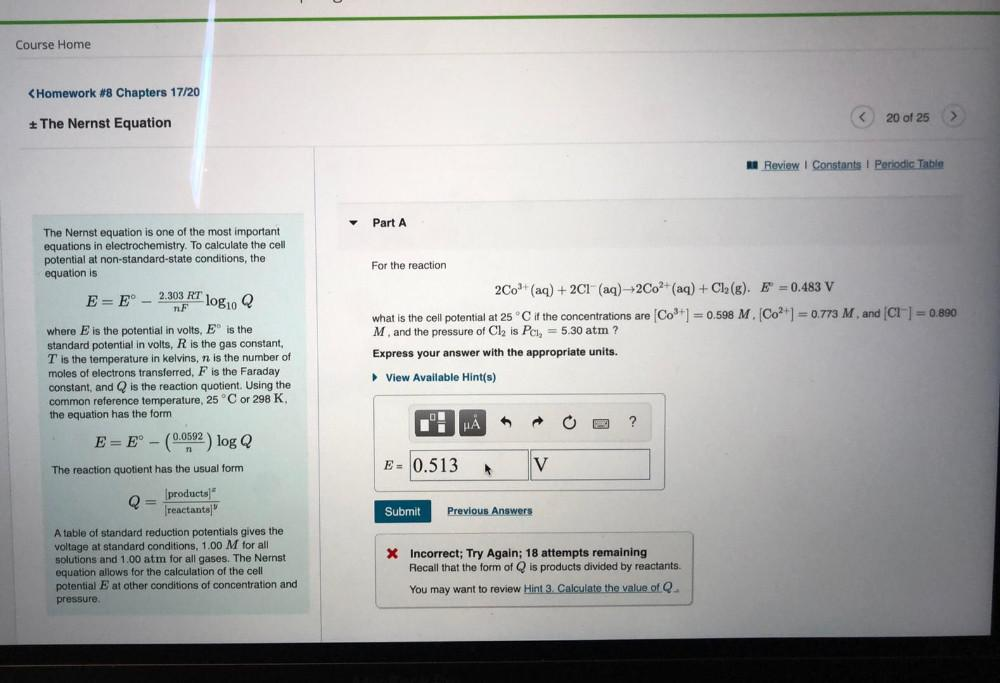Question:

# The Nernst equation is one of the most important equations

Last updated: 7/28/2022The Nernst equation is one of the most important equations in electrochemistry. To calculate the cell potential at non-standard-state conditions, the equation is E = E˚-(2.303 RT/nF) * log10 where E is the potential in volts, Eº is the standard potential in volts, R is the gas constant, T is the temperature in kelvins, n is the number of moles of electrons transferred, and F is the Faraday constant, and is the reaction quotient. Using the common reference temperature, 25 °C or 298 K, the equation has the form E = E˚-[(0.0592)/n] log Q The reaction quotient has the usual form Q =[products]"/[reactants]" A table of standard reduction potentials gives the voltage at standard conditions, 1.00 M for all solutions and 1.00 atm for all gases. The Nernst equation allows for the calculation of the cell potential E at other conditions of concentration and pressure. For the reaction 2Co³+ (aq) + 2Cl-(aq)→2Co²+ (aq) + Cl₂ (g). Eº = 0.483 V what is the cell potential at 25°C if the concentrations are [Co³+] =0.598M, [Co²+]= 0.773M and [Cl-]= 0.890M and the pressure of Cl₂ is PCl2 = 5.30 atm ? Express your answer with the appropriate units.